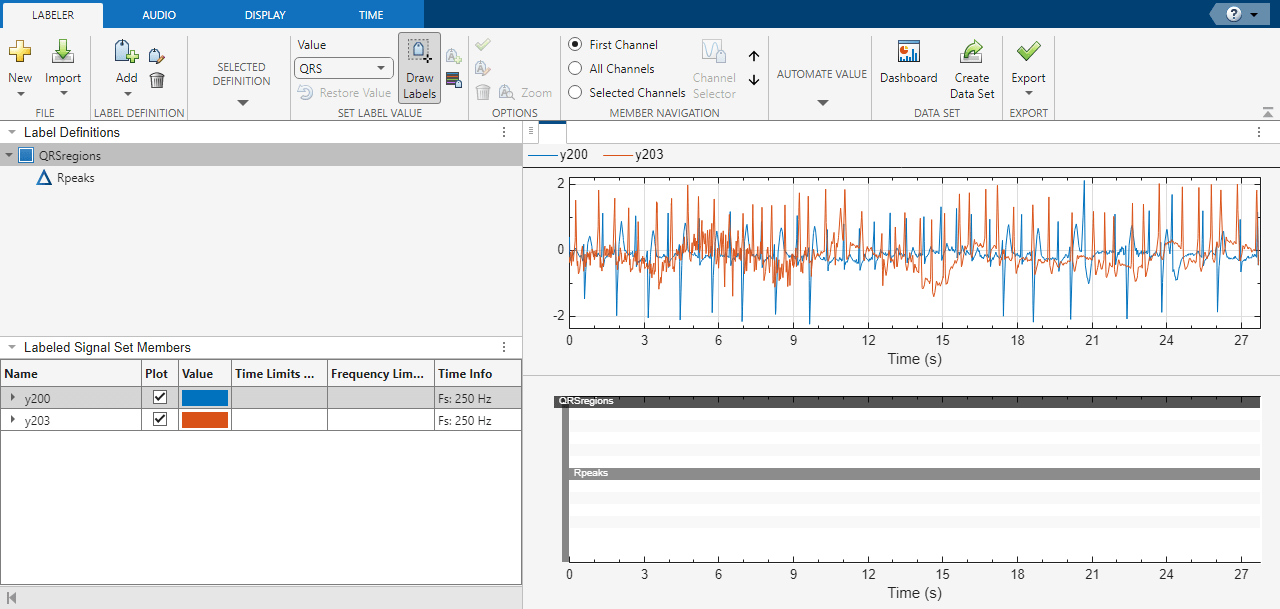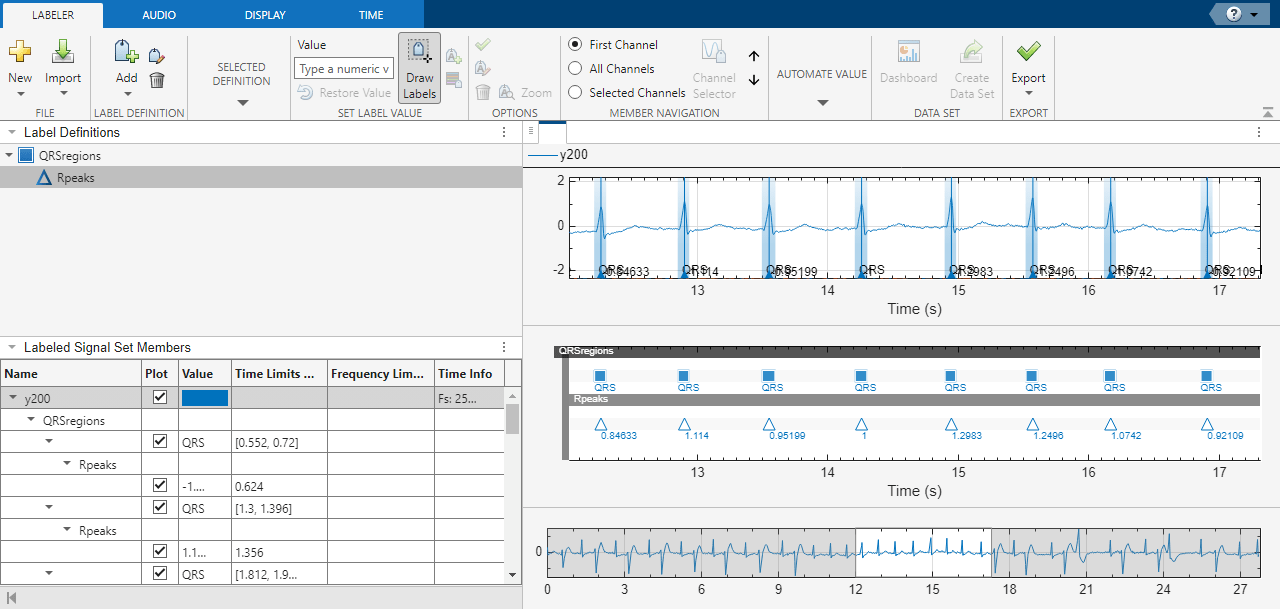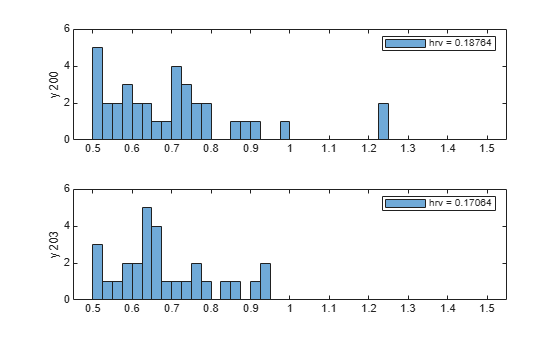Documentation

Label QRS Complexes and R Peaks of ECG Signals Using Deep Network

This example shows how to use custom automated labeling functions in Signal Labeler to label QRS complexes and R peaks of electrocardiogram (ECG) signals. One custom function uses a previously trained recurrent deep learning network to identify and locate the QRS complexes. Another custom function uses a simple peak finder to locate the R peaks. In the example, the network labels the QRS complexes of two signals that are completely independent of the network training and testing process.

The QRS complex, which consists of three deflections in the ECG waveform, reflects the depolarization of the right and left ventricles of the heart. The QRS is also the highest-amplitude segment of the human heartbeat. Study of the QRS complex can help assess the overall health of a person's heart and the presence of abnormalities . In particular, by locating R peaks within the QRS complexes and looking at the time intervals between consecutive peaks, a diagnostician can compute the heart-rate variability of a patient and detect cardiac arrhythmia.

The deep learning network in this example was introduced in Waveform Segmentation Using Deep Learning, where it was trained using ECG signals from the publicly available QT Database  . The data consists of roughly 15 minutes of ECG recordings from a total of 105 patients, sampled at 250 Hz. To obtain each recording, the examiners placed two electrodes on different locations on a patient's chest, which resulted in a two-channel signal. The database provides signal region labels generated by an automated expert system . The added labels make it possible to use the data to train a deep network. See Waveform Segmentation Using Deep Learning for more details.

Load, Resample, and Import Data into Signal Labeler

The signals labeled in this example are from the MIT-BIH Arrhythmia Database . Each signal in the database was sampled at 360 Hz and was annotated by two cardiologists, allowing for verification of the results.

Load two of the MIT database signals, corresponding to records 200 and 203. Resample the signals to 250 Hz, the sample rate of the QT Database data.

y200 = resample(ecgsig,25,36);

y203 = resample(ecgsig,25,36);

Start Signal Analyzer and drag the signals to the Signal table. Select the signals. Add time information: on the Analyzer tab, click Time Values, select Sample Rate and Start Time, and specify a sample rate of 250 Hz. On the Analyzer tab, click Label. The signals appear in the Labeled Signal Set browser.

Define Labels

Define labels to attach to the signals.

1. Define a categorical region-of-interest (ROI) label for the QRS complexes. Click Add Definition on the Label tab. Specify the Label Name as QRSregions, select a LabelType of ROI, enter the Data Type as categorical, and add two Categories, QRS and n/a, each on its own line.

2. Define a numerical point label for the R peaks and set it as a sublabel of QRSregions. Click QRSregions in the Label Definitions browser to select it. Click Add Definition ▼ and select Add sublabel definition. Specify the Label Name as Rpeaks, select a LabelType of Point, and enter the Data Type as numeric.Create Custom Autolabeling Functions

Create two custom functions, one to locate and label the QRS complexes and another to locate and label the R peak within each QRS complex. (Code for the findQRS, computeFSST, p2qrs, and findRpeaks functions appears later in the example.) To create each function, in the Analyzer tab, click Automate Value ▼ and select Add Custom Function. Signal Labeler shows a dialog box asking for the name, description, and label type of the function to add.

1. For the function that locates the QRS complexes, enter findQRS in the Name field and select ROI as the Label Type. You can leave the Description field empty or you can enter your own description.

2. For the function that locates the R peaks, enter findRpeaks in the Name field and select Point as the Label Type. You can leave the Description field empty or you can enter your own description.

If you already have written the functions, and the functions are in the current folder or in the MATLAB® path, Signal Labeler adds the functions to the gallery. If you have not written the functions, Signal Labeler opens blank templates in the Editor for you to type or paste the code. Save the files. The functions appear in the gallery.Label QRS Complexes and R Peaks

Find and label the QRS complexes of the input signals.

1. In the Labeled Signal Set browser, select the check box next to y200.

2. Select QRSregions in the Label Definitions browser.

3. On the Automate Value gallery, select findQRS.

4. Click Auto-Label and click OK in the dialog box that appears.

Signal Labeler locates and labels the QRS complexes for all signals but displays only those of the signal whose check box you selected. The QRS complexes appear as shaded regions in the plot and in the label viewer axes. Activate the panner by clicking Panner on the Display tab and zoom in on a region of the labeled signal.Find and label the R peaks corresponding to the QRS complexes.

1. Select Rpeaks in the Label Definitions browser.

2. Go back to the Label tab. On the Automate Value gallery, select findRpeaks.

3. Click Auto-Label and click OK in the dialog box that appears.

The labels and their numeric values appear in the plot and in the label viewer axes.Export Labeled Signals and Compute Heart-Rate Variability

Export the labeled signals to compare the heart-rate variability for each patient. On the Label tab, click Save Labels. In the dialog box that appears, give the name heartrates to the labeled signal set. Click OK to return to Signal Analyzer. In the Signal table, select heartrates and right-click to export it to a file called HeartRates.mat.

Load the labeled signal set. For each signal in the set, compute the heart-rate variability as the standard deviation of the time differences between consecutive heartbeats. Plot a histogram of differences and display the heart-rate variability.

nms = getMemberNames(heartrates);

for k = 1:heartrates.NumMembers

v = getLabelValues(heartrates,k,{'QRSregions','Rpeaks'});

hr = diff(cellfun(@(x)x.Location,v));

subplot(2,1,k)
histogram(hr,0.5:.025:1.5)
legend(['hrv = ' num2str(std(hr))])
ylabel(nms(k))
ylim([0 6])

endfindQRS Function: Find QRS Complexes

The findQRS function finds and labels the QRS complexes of the input signals.

The function uses two auxiliary functions, computeFSST and p2qrs. (Code for both auxiliary functions appears later in the example.) You can either store the functions in separate files in the same directory or nest them inside findQRS by inserting them before the final end statement.

Between calls to computeFSST and p2qrs, findQRS uses the classify function and the trained deep network net to identify the QRS regions. Before calling classify, findQRS converts the data into the format expected by net, as explained in Waveform Segmentation Using Deep Learning:

• Each signal must be sampled at 250 Hz and partitioned into a stack of 2-by-N cell arrays, where each row corresponds to a channel and N is a multiple of 5000. The actual partitioning and stacking is done in the computeFSST function.

• Each of the resampled MIT signals has 6945 samples, a number that is not a multiple of 5000. To keep all the data in each signal, pad the signal with random numbers. Later in the process, the p2qrs function labels the random numbers as not belonging to QRS complexes and discards them.

function [labelVals,labelLocs] = findQRS(x,t,parentLabelVal,parentLabelLoc,varargin)
% This is a template for creating a custom function for automated labeling
%
%  x is a matrix where each column contains data corresponding to a
%  channel. If the channels have different lengths, then x is a cell array
%  of column vectors.
%
%  t is a matrix where each column contains time corresponding to a
%  channel. If the channels have different lengths, then t is a cell array
%  of column vectors.
%
%  parentLabelVal is the parent label value associated with the output
%  sublabel or empty when output is not a sublabel.
%  parentLabelLoc contains an empty vector when the parent label is an
%  attribute, a vector of ROI limits when parent label is an ROI or a point
%  location when parent label is a point.
%
%  labelVals must be a column vector with numeric, logical or string output
%  values.
%  labelLocs must be an empty vector when output labels are attributes, a
%  two column matrix of ROI limits when output labels are ROIs, or a column
%  vector of point locations when output labels are points.

labelVals = [];
labelLocs = [];

Fs = 250;

for kj = 1:size(x,2)

sig = x(:,kj);

% Create 10000-sample signal expected by the deep network

sig = [sig;randn(10000-length(sig),1)/100]';

% Resize input and compute synchrosqueezed Fourier transforms

mitFSST = computeFSST(sig,Fs);

% Use trained network to predict which points belong to QRS regions

netPreds = classify(net,mitFSST,'MiniBatchSize',50);

% Convert stack of cell arrays into a single vector

Location = [1:length(netPreds{1}) length(netPreds{1})+(1:length(netPreds{2}))]';
Value = [netPreds{1} netPreds{2}]';

% Label QRS complexes as regions of interest and discard non-QRS data

[Locs,Vals] = p2qrs(table(Location,Value));

labelVals = [labelVals;Vals];
labelLocs = [labelLocs;Locs/Fs];

end

% Insert computeFSST and p2qrs here if you want to nest them inside
% queryQRS instead of including them as separate functions in the folder.

end

computeFSST Function: Resize Input and Compute Synchrosqueezed Fourier Transforms

This function reshapes the input data into the form expected by net and then uses the fsst function to compute the Fourier synchrosqueezed transform (FSST) of the input. In Waveform Segmentation Using Deep Learning, the network performs best when given as input a time-frequency map of each training or testing signal. The FSST results in a set of features particularly useful for recurrent networks because the transform has the same time resolution as the original input. The function:

• Specifies a Kaiser window of length 128 to provide adequate frequency resolution.

• Extracts data over the frequency range from 0.5 Hz to 40 Hz.

• Subtracts the mean of each signal and divides by the standard deviation.

• Treat the real and imaginary parts of the FSST as separate features.

function signalsFsst = computeFSST(xd,Fs)

targetLength = 5000;
signalsOut = {};

for sig_idx = 1:size(xd,1)

current_sig = xd(sig_idx,:)';

% Compute the number of targetLength-sample chunks in the signal
numSigs = floor(length(current_sig)/targetLength);

% Truncate to a multiple of targetLength
current_sig = current_sig(1:numSigs*targetLength);

% Create a matrix with as many columns as targetLength signals
xM = reshape(current_sig,targetLength,numSigs);

% Vertically concatenate into cell arrays
signalsOut = [signalsOut; mat2cell(xM.',ones(numSigs,1))];

end

signalsFsst = cell(size(signalsOut));

for idx = 1:length(signalsOut)

[s,f] = fsst(signalsOut{idx},Fs,kaiser(128));

% Extract data over the frequency range from 0.5 Hz to 40 Hz
f_indices = (f > 0.5) & (f < 40);
signalsFsst{idx}= [real(s(f_indices,:)); imag(s(f_indices,:))];

signalsFsst{idx} = (signalsFsst{idx}-mean(signalsFsst{idx},2)) ...
./std(signalsFsst{idx},[],2);

end

end

p2qrs Function: Label QRS Complexes as Regions of Interest

The deep network outputs a categorical array that labels every point of the input signal as belonging a P region, a QRS complex, a T region, or to none of those. This function converts those point labels to QRS region-of-interest labels.

• To perform the conversion, the function assigns integer numerical values to the categories and uses the findchangepts function to find the points where the numerical array changes value.

• Each of those changepoints is the left endpoint of a categorical region, and the point that precedes it in the array is the right endpoint of the preceding region.

• The algorithm adds 1e-6 to the right endpoints to prevent one-sample regions from having zero duration.

• The df parameter selects as regions of interest only those QRS complexes whose duration is greater than df samples.

function [locs,vals] = p2qrs(k)

fc = 1e-6;
df = 20;

ctgs = categories(k.Value);
levs = 1:length(ctgs);
for jk = levs
cat2num(k.Value == ctgs{jk}) = levs(jk);
end
chpt = findchangepts(cat2num,'MaxNumChanges',length(cat2num));
locs = [[1;chpt'] [chpt'-1;length(cat2num)]+fc];

vals = categorical(cat2num(locs(:,1))',levs,ctgs);
locs = locs+round(k.Location(1))-1;

qrs = find(vals=='QRS' & diff(locs,[],2)>df);

vals = categorical(string(vals(qrs)),["QRS" "n/a"]);

locs = locs(qrs,:);

end

findRpeaks Function: Find R Peaks

This function locates the most prominent peak of the QRS regions of interest found by findQRS. The function applies the MATLAB® islocalmax function to the absolute value of the signal in the intervals located by findQRS.

function [labelVals,labelLocs] = findRpeaks(x,t,parentLabelVal,parentLabelLoc,varargin)

Fs = 250;

if isempty(t)
t = (0:length(x)-1)'/Fs;
end

labelVals = zeros(size(parentLabelLoc,1),1);
labelLocs = zeros(size(parentLabelLoc,1),1);

for kj = 1:size(parentLabelLoc,1)
tvals = t>=parentLabelLoc(kj,1) & t<=parentLabelLoc(kj,2);
ti = t(tvals);
xi = x(tvals);
lc = islocalmax(abs(xi),'MaxNumExtrema',1);
labelVals(kj) = xi(lc);
labelLocs(kj) = ti(lc);
end

end

References

 Laguna, Pablo, Raimon Jané, and Pere Caminal. "Automatic detection of wave boundaries in multilead ECG signals: Validation with the CSE database." Computers and Biomedical Research. Vol. 27, No. 1, 1994, pp. 45–60.

 Goldberger, Ary L., Luis A. N. Amaral, Leon Glass, Jeffery M. Hausdorff, Plamen Ch. Ivanov, Roger G. Mark, Joseph E. Mietus, George B. Moody, Chung-Kang Peng, and H. Eugene Stanley. "PhysioBank, PhysioToolkit, and PhysioNet: Components of a New Research Resource for Complex Physiologic Signals." Circulation. Vol. 101, No. 23, 2000, pp. e215–e220. [Circulation Electronic Pages: http://circ.ahajournals.org/content/101/23/e215.full].

 Laguna, Pablo, Roger G. Mark, Ary L. Goldberger, and George B. Moody. "A Database for Evaluation of Algorithms for Measurement of QT and Other Waveform Intervals in the ECG." Computers in Cardiology. Vol. 24, 1997, pp. 673–676.

 Moody, George B., and Roger G. Mark. "The impact of the MIT-BIH Arrhythmia Database." IEEE Engineering in Medicine and Biology Magazine. Vol. 20, No. 3, May–June 2001, pp. 45–50.